A heap of paddy is in the form of a right circular cone whose diameter is 4.2 m and height 2.8 m if the heap is to be covered exactly by a canvas to protect it from rain the find the area of the canvas neede.

# A heap of paddy is in the form of a right circular cone whose diameter is 4.2 m and height 2.8 m if the heap is to be covered exactly by a canvas to protect it from rain the find the area of the canvas neede.

1. A
23.1
2. B
78.2
3. C
98.2
4. D
88.5

Fill Out the Form for Expert Academic Guidance!l

+91

Live ClassesBooksTest SeriesSelf Learning

Verify OTP Code (required)

### Solution:

Required area of canvas is equal to curved surface area of cone.
diameter = 4.2 m, radius  = 2.1 m, height = 2.8 m  Let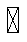be the slant height of cone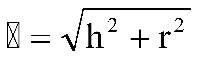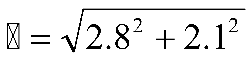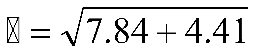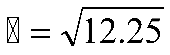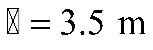Curved surface area of cone =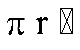Hence option (1) is correct.

## Related content

 Area of Square Area of Isosceles Triangle Pythagoras Theorem Triangle Formula Perimeter of Triangle Formula Area Formulae Volume of Cone Formula Matrices and Determinants_mathematics Critical Points Solved Examples Type of relations_mathematics+91

Live ClassesBooksTest SeriesSelf Learning

Verify OTP Code (required)